# 基于多元统计分析的城区经济发展研究——以武汉市为例Research on Urban Economic Development Based on Multivariate Statistical Analysis—Take Wuhan as an Example

DOI: 10.12677/SA.2020.94065, PDF, HTML, XML, 下载: 67  浏览: 130

Abstract: Since the “rise of central China” plan, the development of Wuhan city has been one of the key na-tional concerns. After years of development, Wuhan’s economy has made remarkable achievements, but there are still some differences in the economic development of various urban areas. Because of the large-scale outbreak of covid-19, the economic development of Wuhan has been impacted. In view of this, this paper studies the economic development of each urban area in Wuhan. Firstly, it selects appropriate index variables, and then objectively compares the ranking of urban economic development in Wuhan based on the scores of principal component analysis and factor analysis, and gives a comprehensive evaluation model. Then cluster analysis and discriminant analysis are used to study the structure of economic development, and indicate the current situation of economic de-velopment in Wuhan city. Finally, it provides optimization measures and suggestions for the eco-nomic development of Wuhan.

1. 引言

2. 材料与方法

2.1. 城区经济发展研究变量体系的设立原则

1) 代表性。对城区经济发展进行评价一定要体现代表性，选取的变量要与经济中能够区分不同城区经济发展水平，并且可以反映问题具有代表性的变量。

2) 可行性。选取指标时，要充分考虑所选取的变量是否适合用来定量分析，以确保分析的可行性和分析结果的客观性。

3) 可获得性。选取变量时要考虑所需数据的来源，在遵循代表性和可行性的基础上，选择容易通过统计年鉴、单位部门等有关途径获得准确数据的变量。

4) 数据正确性。数据最好源自国家或各级政府部门公开出版的报告、发布的统计资料，以确保数据的准确性。

2.2. 武汉市城区经济发展研究变量的选取

1) 全社会固定资产投资额：投资额越高，表示用来满足人民生活生产的投资活动越多。

2) 社会消费品零售总额：用来反映一定时期内人民物质文化生活水平的提高情况，是反映社会商品购买力、零售市场规模和经济活跃度的重要指标。

3) 从业人员平均数：指报告期内平均每天拥有的从业人员数，包括全部职工、再就业的离退休人员、个体从业人员等，它是一定时期内全部劳动力资源的实际利用情况的一个反应。

4) 分区生产总值：反应的是国内生产总值在城区范围内一定时期里生产活动的最终成果，从不同的侧面反映某地区的经济规模和经济总量情况。

5) 城镇居民人均可支配收入：反映居民可以用来安排家庭日常生活的全部现金收入，是反映居民收入状况的一个重要指标。

6) 分区公共财政总收入：地区财政参与社会产品分配所取得的收入，是地区政府行使相关职能的财力保障。

7) 主营业务收入：通常指企业的经常性的、主要业务所产生的基本收入，是反映企业盈利能力的一个重要指标。

8) 城镇居民人均消费支出：指城镇居民在购买商品和劳务活动两方面的支出，往往用来衡量居民的消费支出情况。

9) 工业总产值：指以货币形式表现的工业企业在报告期内生产的工业产品总量，是衡量一定时间内工业生产水平和规模状况的重要指标。

2.3. 城区经济发展研究的方法

2.3.1. 主成分分析法

① 数学模型

${X}_{1},\text{\hspace{0.17em}}{X}_{2},\text{\hspace{0.17em}}{X}_{3},\text{\hspace{0.17em}}\cdots ,\text{\hspace{0.17em}}{X}_{p}$ 表示P个原始指标，用这p个指标对n个样品进行评价，那么共有np个数据，在实际问题的研究中，通常这p个原始变量会具有不同的量纲，为了消除量纲的影响，进行主成分分析之前，需要将数据进行标准化，设标准化后的变量为 $\text{\hspace{0.17em}}{x}_{1},\text{\hspace{0.17em}}{x}_{2},\text{\hspace{0.17em}}\cdots ,{x}_{p}$

$\left\{\begin{array}{l}{y}_{1}={w}_{11}{x}_{1}+{w}_{12}{x}_{2}+\cdots +{w}_{1p}{x}_{p}\\ {y}_{2}={w}_{21}{x}_{1}+{w}_{22}{x}_{2}+\cdots +{w}_{2p}{x}_{p}\\ ⋮\\ {y}_{p}={w}_{p1}{x}_{1}+{w}_{p2}{x}_{2}+\cdots +{w}_{pp}{x}_{p}\end{array}$

1) ${y}_{i}$${y}_{j}$ 相互独立

2) $\mathrm{var}\left({y}_{1}\right)\ge \mathrm{var}\left({y}_{2}\right)\ge \cdots \ge \mathrm{var}\left( y p \right)$

3) ${w}_{i1}^{2}+{w}_{i2}^{2}+\cdots {w}_{ip}^{2}=1,\text{\hspace{0.17em}}i=\text{\hspace{0.17em}}1,\text{\hspace{0.17em}}2,\text{\hspace{0.17em}}\cdots ,\text{\hspace{0.17em}}p$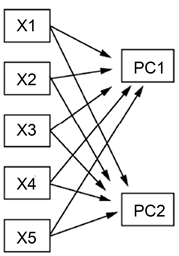Figure 1. PCA model (variable x1-x5 maps the PC1, PC2)

② 主成分的选取

$\underset{i=1}{\overset{m}{\sum }}{\alpha }_{i}=\frac{\underset{i=1}{\overset{m}{\sum }}{\lambda }_{i}}{\underset{i=1}{\overset{p}{\sum }}{\lambda }_{i}}\left(m 表示前m个主成分的累计方差贡献率

③ 主成分得分

2.3.2. 因子分析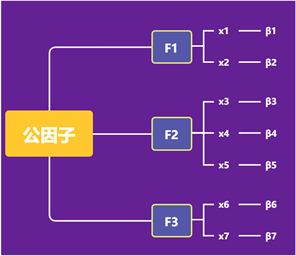Figure 2. Factor analysis

$\left\{\begin{array}{l}{x}_{1}={\alpha }_{11}{f}_{1}+\alpha 12{f}_{2}+\cdots +{\alpha }_{1k}{f}_{k}+{\beta }_{1}\\ {x}_{2}={\alpha }_{12}{f}_{1}+{\alpha }_{22}{f}_{2}+\cdots +{\alpha }_{2k}{f}_{k}+{\beta }_{2}\\ ⋮\\ xp={\alpha }_{p1}{f}_{1}+{\alpha }_{p2}{f}_{2}+\cdots +{\alpha }_{pk}{f}_{k}+{\beta }_{p}\end{array}$

2.3.3. 聚类分析

2.3.4. 判别分析

3. 结果与分析

3.1. 主成分分析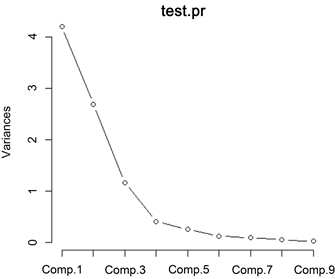Figure 3. Scree plot

$F1=0.201{x}_{1}-0.373{x}_{2}+0.324{x}_{3}-0.387{x}_{4}-0.381{x}_{5}-0.371{x}_{6}+0.353{x}_{7}-0.341{x}_{8}+0.206{x}_{9}$ ;

$F2=0.698{x}_{1}+0.153{x}_{3}+0.193{x}_{3}+0.143{x}_{4}+0.193{x}_{6}+0.142{x}_{7}-0.104{x}_{8}-0.600{x}_{9}$ ;

$F3=0.114{x}_{1}-0.534{x}_{3}-0.436{x}_{5}-0.482{x}_{7}-0.534{x}_{8}$

$Y=\left(F1*0.5900+F2*0.1521+F3*0.1246\right)/0.8667$Table 5. Scores of principal component and comprehensive ranking

3.2. 因子分析

3.2.1. 利用SPSS进行因子分析Table 6. KMO and Barlett testTable 8. Total variance interpretation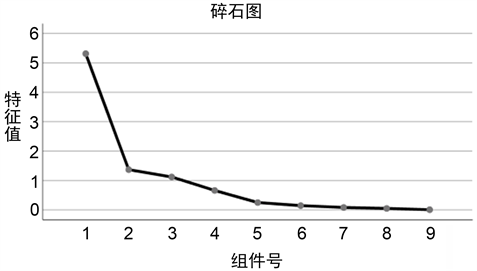Figure 4. Scree plot

Table 9. Rotating component matrixTable 10. Component score coefficient matrix

$F={H}^{\prime }X-1{B}^{\prime }$ 计算其因子得分，其中 $F=\left(F1,\text{\hspace{0.17em}}\text{\hspace{0.17em}}F2,\text{\hspace{0.17em}}\text{\hspace{0.17em}}F3\right)$，Fi为第i个公因子的得分，以各因子的加权方差贡献率作为权重，最后得到武汉市城区经济发展研究的综合评价公式为：

$Y=\left(F1*0.59004+F2*0.15209+F3*0.12455\right)/0.86668$Table 11. Factor score and comprehensive score

3.2.2. 利用R软件进行因子分析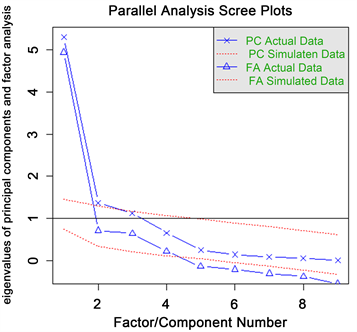Figure 5. Factor analysis

fa函数中，第一个参数为数据，第二个参数说明要保留3个主成分，第三个参数为旋转方法，为none，即不进行主成分旋转，第四个参数表示提取公因子的方法为最大似然法。上图表明输出结果与主成分输出结果基本一致，并可看出该数据选取三个主成分解释了原始变量80%的方差。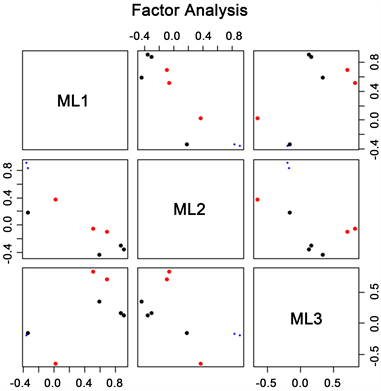Figure 6. Data visualization

3.3. 结果检验

**.在0.01级别(双尾)，相关性显著。

3.4. 聚类分析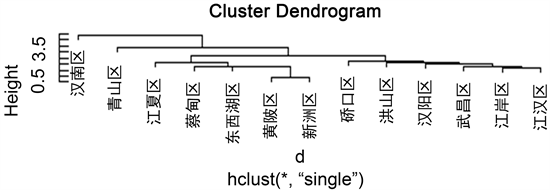Figure 7. Shortest distance method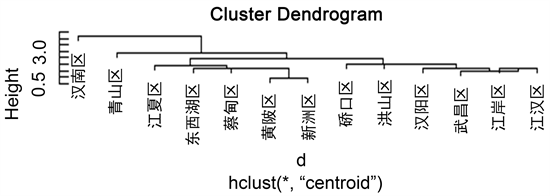Figure 8. Centroid method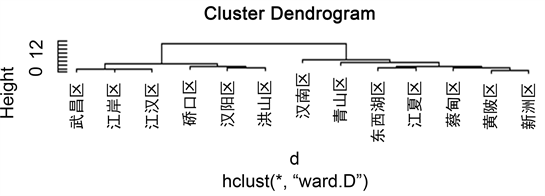Figure 9. Ward method

3.5. 判别分析Table 19. Distance discrimination resultsTable 20. Bayes discrimination results

4. 讨论

4.1. 总结

4.2. 政策建议

1) 对城区经济较发达地区，合理优化其产业结构，推进城区产业均衡，提高江汉区、江岸区、洪山区、武昌区的工业发展水平，同时应当注重科技创新，在武汉高新技术开发区、武汉光谷等国家级高新技术产业园区的快速发展中，充分利用其科技优势资源，重点培养和发展生物制药、互联网、光缆通讯、精密仪器制造等产业。

2) 对城区经济一般发达地区，逐步缩小城区发展不平衡，全面协调可持续发展。这类城区多为远郊城区，应当充分发挥中心城区经济发展的辐射作用，带动远郊城区的经济发展，引导城区经济逐步走向一体化，因此政府应加快推进工业化进程，把远城区的发展看作是武汉新型工业化和新城镇化的战略支点，同时要促进消费，提高经济活跃度。

3) 打造城区特色，借助城区优势，发展城区重点经济。市政府应当提高对各城区自身发展的条件和可以利用的资源的认识，建立不同城区在不同资源领域、不同技术方向上的竞争优势。如青山区继续推动武钢、武石化升级改造，建设绿色低碳循环发展的一流生态化工园区。武昌区进一步发展东湖生态旅游风景区，力求打造国际知名、国内一流的文化旅游生态风景名胜区等。另外要加大对经济实力较弱的汉南区的资金投入，扶植其优势产业，带动其经济发展。总之各城区应当相互借鉴、取长补短，将自己的优势产业做大做强，更好地促进全市经济稳步快速地发展 。

  文乐. 基于多元统计分析的武汉市城区经济发展研究[D]: [硕士学位论文]. 武汉: 华中师范大学, 2016.  樊长科, 吴雨. 广西各地市区域经济发展水平分析——基于系统繁类法和主成分分析法[J]. 安徽农业科学, 2010, 38(14): 7620-7622.  杨曼琳. 基于主成分分析法的区域经济活力影响因素[J]. 中国科技信息, 2020(12): 106+108.  姜天龙, 刘子宜, 单魁贤. 吉林省县域经济社会发展的综合评价及分析——基于主成分分析法[J]. 农村经济与科技, 2014, 25(10): 120-122.  张小爱. 河北省区域经济的统计分析[J]. 统计与管理, 2018(12): 39-42.  刘孟轩. 宏观经济背景下统计分析相关问题及其对策研究[J]. 现代交际, 2020(10): 42-43.